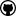org.dllearner.core

## Class AbstractLearningProblem<T extends Score,V extends org.semanticweb.owlapi.model.OWLObject,W extends EvaluatedHypothesis<V,T>>

• ### Constructor Summary

Constructors
Constructor and Description
`AbstractLearningProblem()`
`AbstractLearningProblem(AbstractReasonerComponent reasoner)`
Constructs a learning problem using a reasoning service for querying the background knowledge.
• ### Method Summary

All Methods
Modifier and Type Method and Description
`void` `changeReasonerComponent(AbstractReasonerComponent reasoner)`
Method to exchange the reasoner underlying the learning problem.
`T` `computeScore(V hypothesis)`
Computes the `Score` of a given hypothesis with respect to this learning problem.
`abstract T` ```computeScore(V hypothesis, double noise)```
Computes the `Score` of a given hypothesis with respect to this learning problem.
`W` `evaluate(V hypothesis)`
Evaluates the hypothesis by computing the score and returning an evaluated hypothesis of the correct type (ClassLearningProblem returns EvaluatedDescriptionClass instead of generic EvaluatedDescription).
`W` ```evaluate(V hypothesis, double noise)```
Evaluates the hypothesis by computing the score and returning an evaluated hypothesis of the correct type (ClassLearningProblem returns EvaluatedDescriptionClass instead of generic EvaluatedDescription).
`double` `getAccuracyOrTooWeak(V object)`
This method returns a value, which indicates how accurate a hypothesis solves a learning problem.
`abstract double` ```getAccuracyOrTooWeak(V hypothesis, double noise)```
This method computes the accuracy and returns -1 instead of the accuracy if the accuracy of the hypothesis is below the given threshold and the accuracy of all more special w.r.t.
`AbstractReasonerComponent` `getReasoner()`
Implementations of learning problems can use this class variable to perform reasoner operations.
`void` `setReasoner(AbstractReasonerComponent reasoner)`
• ### Methods inherited from class org.dllearner.core.AbstractComponent

`isInitialized`
• ### Methods inherited from class java.lang.Object

`equals, getClass, hashCode, notify, notifyAll, toString, wait, wait, wait`
• ### Methods inherited from interface org.dllearner.core.Component

`init`
• ### Constructor Detail

• #### AbstractLearningProblem

`public AbstractLearningProblem()`
• #### AbstractLearningProblem

`public AbstractLearningProblem(AbstractReasonerComponent reasoner)`
Constructs a learning problem using a reasoning service for querying the background knowledge. It can be used for evaluating solution candidates.
Parameters:
`reasoner` - The reasoning service used as background knowledge.
• ### Method Detail

• #### changeReasonerComponent

`public void changeReasonerComponent(AbstractReasonerComponent reasoner)`
Method to exchange the reasoner underlying the learning problem. Implementations, which do not only use the provided reasoning service class variable, must make sure that a call to this method indeed changes the reasoning service.
Parameters:
`reasoner` - New reasoning service.
• #### computeScore

`public T computeScore(V hypothesis)`
Computes the `Score` of a given hypothesis with respect to this learning problem. This can (but does not need to) be used by learning algorithms to measure how good the hypothesis fits the learning problem. Score objects are used to store e.g. covered examples, accuracy etc., so often it is more efficient to only create score objects for promising hypotheses.
Parameters:
`hypothesis` - A hypothesis (as solution candidate for this learning problem).
Returns:
A `Score` object.
• #### computeScore

```public abstract T computeScore(V hypothesis,
double noise)```
Computes the `Score` of a given hypothesis with respect to this learning problem. This can (but does not need to) be used by learning algorithms to measure how good the hypothesis fits the learning problem. Score objects are used to store e.g. covered examples, accuracy etc., so often it is more efficient to only create score objects for promising hypotheses.
Parameters:
`hypothesis` - A hypothesis (as solution candidate for this learning problem).
`noise` - the (approximated) value of noise within the examples
Returns:
A `Score` object.
• #### evaluate

`public W evaluate(V hypothesis)`
Evaluates the hypothesis by computing the score and returning an evaluated hypothesis of the correct type (ClassLearningProblem returns EvaluatedDescriptionClass instead of generic EvaluatedDescription).
Parameters:
`hypothesis` - Hypothesis to evaluate.
Returns:
an evaluated hypothesis
• #### evaluate

```public W evaluate(V hypothesis,
double noise)```
Evaluates the hypothesis by computing the score and returning an evaluated hypothesis of the correct type (ClassLearningProblem returns EvaluatedDescriptionClass instead of generic EvaluatedDescription).
Parameters:
`hypothesis` - Hypothesis to evaluate.
`noise` - the (approximated) value of noise within the examples
Returns:
an evaluated hypothesis
• #### getAccuracyOrTooWeak

`public double getAccuracyOrTooWeak(V object)`
This method returns a value, which indicates how accurate a hypothesis solves a learning problem. There can be different ways to compute accuracy depending on the type of learning problem and other factors. However, all implementations are required to return a value between 0 and 1, where 1 stands for the highest possible accuracy and 0 for the lowest possible accuracy.
Returns:
A value between 0 and 1 indicating the quality (of a hypothesis). or -1 as described above.
• #### getAccuracyOrTooWeak

```public abstract double getAccuracyOrTooWeak(V hypothesis,
double noise)```
This method computes the accuracy and returns -1 instead of the accuracy if
1. the accuracy of the hypothesis is below the given threshold and
2. the accuracy of all more special w.r.t. subsumption hypotheses is below the given threshold.
This is used for efficiency reasons, i.e. -1 can be returned instantly if it is clear that the hypothesis and all its refinements are not sufficiently accurate.
Returns:
A value between 0 and 1 indicating the quality (of a hypothesis) or -1 as described above.
• #### getReasoner

`public AbstractReasonerComponent getReasoner()`
Implementations of learning problems can use this class variable to perform reasoner operations.
• #### setReasoner

```@Autowired(required=false)
public void setReasoner(AbstractReasonerComponent reasoner)```DL-Learner is licenced under the terms of the GNU General Public License.